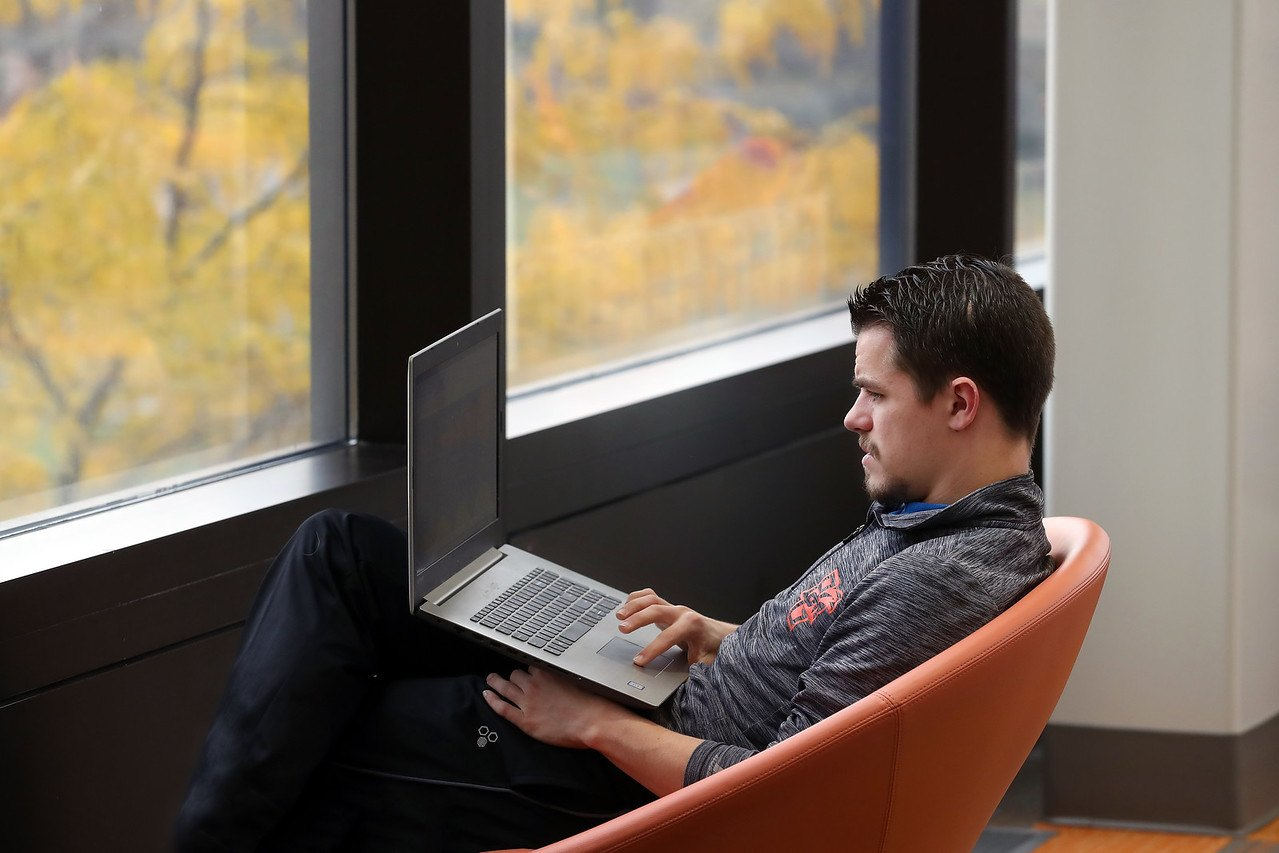The Applied Mathematics and Scientific Computational program is part of the Department of Mathematics and Statistics in the BGSU College of Arts and Sciences.• Masters Available

Masters of Arts in

# Applied Mathematics and Scientific Computation

The BGSU master’s program in applied mathematics and scientific computing is built upon rigorous training of mathematics theory and programming practice. At its core are the design of approximation algorithms and the development of error estimation methodologies for the numerical solutions of partial differential equations and other mathematical models.

Students in this program acquire rigorous training in real analysis and functional analysis, while having access to other branches of math courses as well. They also have a breadth of mathematical modeling through the applied math curriculum. Students will have significant practical computational experiences, which will be of great value in further research or an industrial setting.

Students will also find opportunities to apply mathematics in geophysics, finance, materials science, fluid dynamics, mathematical biology, physics and chemistry.

## The power of modern computing applied to complex real-world problems

The BGSU Department of Mathematics and Statistics enjoys active and collegial relationships with the other science and engineering departments. Students will find a liberal arts atmosphere in a University setting conducive to interdisciplinary education and basic research.

Highlights of research projects being conducted at BGSU by members for the Applied Mathematics faculty in collaboration with researchers from other departments include:

Numerical Partial Differential Equations

Computational methodology combined with convergence theory, including: the immersed finite element method, finite volume methods, discontinuous Galerkin methods, and adaptive methods based on numerical smoothness and super-convergence theory.

Numerical Smoothness and Optimal Error Estimations

An innovative idea named as numerical smoothness has been proposed and developed for the error estimation and adaptive error control for the numerical solutions of time-dependent differential equations, ordinary, parabolic or hyperbolic. This idea is parallel to the numerical stability theory, which has been considered as the fundamental theory of numerical analysis, but it has shown clear advantages in both local error control and global error propagation estimation for complex/nonlinear problems and methods.

Inverse Problems

Parameter estimation for partial differential equations, theory and methodology for large-scale ill-posed optimization, iterative spectral decomposition methods, and regularization techniques and simulation of waves and fields.

Multiphase Porous Media Fluid Flow

Theoretical and numerical treatment of mathematical models with applications in petroleum extraction and groundwater contamination remediation.

## Stand Out in courses like

• Real Analysis
• Iterative Solution of Nonlinear Equations in Several Variables
• Biostatistical Methods
• Numerical Analysis of Partial Differential Equations
• Algebraic Problems and Misconceptions

Small classes, active research faculty and a competitive Putnam team.

## Curriculum

The master’s in applied mathematics and scientific computation is a 30-curriculum hour graduate degree. After a required core of coursework, the curriculum is flexible to allow students to pursue research or professional goals.

Graduation is achieved through successful completion of a master's thesis or passing a comprehensive examination. The written comprehensive exam consists of two three-hour exams based on courses in analysis and applied mathematics.

Students choosing the MA in applied mathematics and scientific computing option are expected to have completed courses in linear algebra, advanced calculus and ordinary differential equations and to have experience in a programming languages such as C, C++, Python, Java or Matlab before (or soon after) entry.

Topics studied include boundary value problems, matrix computations, numerical solution of partial differential equations, optimization and approximation theory.

## Go Far in your career

• Computational Genomics
• Population Analytics
• Predictive Complex Systems
• Nanomaterials
• Computational Chemistry for Excited States
• Computational Materials Chemist
• Computational Finance

## Career

Scientific computing has become a "third scientific methodology,” distinct from theory and experimentation. Exploiting this new approach requires (a) sound mathematical training, (b) expertise in the practical aspects of computing and algorithm development and (c) an interdisciplinary, scientific viewpoint which transcends traditional disciplinary boundaries.

The rewards are career options at the leading edge of computational mathematics as new applications are discovered and new careers become available to those with the right skills.

The BLS finds the applied mathematics profession is facing a 33% expansion over the next decade, much faster than other careers.

Medicine, engineering, finance, urban development, biology and prediction are just some of the areas that can benefit from scientific computing. The promise of saving time and money by computing rather than experimenting or testing makes this a lucrative career option.

Conceptualizing and simulating complex problems.Bowling Green State University [BGSU] is accredited by the Higher Learning Commission.  BGSU has been accredited by the Higher Learning Commission since 01/01/1916. The most recent reaffirmation of accreditation was received in 2012 - 2013. Questions should be directed to the Office of Institutional Effectiveness.